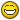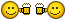Jump to content# Codes to Add Text only and no need leader..

## Recommended Postshai, i need a small help.i have a working lisp routine which gives perpendicular line distance to main line when clicked on a place perpendicular, only thing i want to try and add is to remove the leader that adds and only add text without leader in position where i can click. pls reply if its possible... thanks:)

```(defun c:Test ( / s p c a d)
(if (and (setq s (car (entsel "\nPick a polyline :")))
(or (= (cdr (assoc 0 (entget s))) "LWPOLYLINE")
(alert "Invalid object! Please pick a polyline only.")
)
(setq p (getpoint "\nSpecify point perpendicular to polyline :"))
(setq c (vlax-curve-getclosestpointto s p))
(setq a (angle p c))
(not (grdraw p c 1 -1)) ;; rubber line in red colour.
(setq d (angle '(0. 0. 0.) (vlax-curve-getfirstderiv s (vlax-curve-getparamatpoint s c))))
(or (or (equal (rem (+ d (* pi 0.5)) (+ pi pi)) a 1e-4)
(equal (rem (+ d (* pi 1.5)) (+ pi pi)) a 1e-4)
)
(alert "Picked point is not a perpendicular to picked polyline. <!>")
)
)
(command "_.leader" "_non" p "\\" "" (strcat "CHAINAGE=3+0" (rtos (vlax-curve-getdistatpoint s c) 2 4)) "")
)
(princ)
) (vl-load-com)```

Edited by SLW210
Fixed Code Tags

#### Share this post

##### Share on other sitesTry this :-

```(defun c:Test (/ s p c a d)
(if
(and
(setq s (car (entsel "\nPick a polyline :")))
(or (= (cdr (assoc 0 (entget s))) "LWPOLYLINE")
(alert "Invalid object! Please pick a polyline only.")
)
(setq p (getpoint "\nSpecify point perpendicular to polyline :"))
(setq c (vlax-curve-getclosestpointto s p))
(setq a (angle p c))
(not (grdraw p c 1 -1))
;; rubber line in red colour.
(setq d (angle '(0. 0. 0.)
(vlax-curve-getfirstderiv
s
(vlax-curve-getparamatpoint s c)
)
)
)
(or (or (equal (rem (+ d (* pi 0.5)) (+ pi pi)) a 1e-4)
(equal (rem (+ d (* pi 1.5)) (+ pi pi)) a 1e-4)
)
(alert
"Picked point is not a perpendicular to picked polyline. <!>"
)
)
)
(entmakex
(list
'(0 . "text")
'(100 . "AcDbEntity")
'(67 . 0)
'(370 . 13)
'(100 . "AcDbText")
(cons 10 p)
(cons 40 2.5)			[color="red"]; Change text height here[/color]
(cons 1
(strcat "chainage=3+0"
(rtos (vlax-curve-getdistatpoint s c) 2 4)
)
)
(cons 71 0)
(cons 72 0)
(cons 11 '(0 0 0))
'(100 . "AcDbText")
(cons 73 0)
)
)
)
(princ)
)
(vl-load-com)```

#### Share this post

##### Share on other sitesThank u, it works good

but just want to know if its possible to select the text location manually, as this code lets the text at the point where i click for distance. Like the leader starts and then text location except no leader needed, only text. If possible it would be nice.

This code really helps a lot, thank you so much..

#### Share this post

##### Share on other sitesalso is it possible to continous the command after i select the polyline, like i select the polyline then after were ever i click it gives the distance to it, (like MULTIPLE command).

the current command ask for polyline after every click and length.. thanks:)

#### Share this post

##### Share on other sitesCommand is continuous in this code as per your requirement:

```(defun c:Test (/ s p c a d tp)
(if
(and (setq s (car (entsel "\nPick a polyline :")))
(or (= (cdr (assoc 0 (entget s))) "LWPOLYLINE")
(alert "Invalid object! Please pick a polyline only.")
)
)
(while
(and
(setq
p (getpoint "\nSpecify point perpendicular to polyline :")
)
(setq c (vlax-curve-getclosestpointto s p))
(setq a (angle p c))
(not (grdraw p c 1 -1))
;; rubber line in red colour.
(setq d (angle	'(0. 0. 0.)
(vlax-curve-getfirstderiv
s
(vlax-curve-getparamatpoint s c)
)
)
)
(or
(or (equal (rem (+ d (* pi 0.5)) (+ pi pi)) a 1e-4)
(equal (rem (+ d (* pi 1.5)) (+ pi pi)) a 1e-4)
)
(alert
"Picked point is not a perpendicular to picked polyline. <!>"
)
)
(setq tp (getpoint "\nSpecify Point for Text : "))
)
(entmakex
(list
'(0 . "text")
'(100 . "AcDbEntity")
'(67 . 0)
'(370 . 13)
'(100 . "AcDbText")
(cons 10 tp)
(cons 40 2.5)		; Change text height here
(cons 1
(strcat "chainage=3+0"
(rtos (vlax-curve-getdistatpoint s c) 2 4)
)
)
(cons 71 0)
(cons 72 0)
(cons 11 '(0 0 0))
'(100 . "AcDbText")
(cons 73 0)
)
)
)
)
(princ)
)
(vl-load-com)```

#### Share this post

##### Share on other sitesalso is it possible to continous the command after i select the polyline, like i select the polyline then after were ever i click it gives the distance to it, (like MULTIPLE command).

the current command ask for polyline after every click and length.. thanks:)

I've updated code above for continuous operation, check it

#### Share this post

##### Share on other sitesThank you so much. It works perfectly...i didn't know how to change the code to simple text sting, thanks so much...

#### Share this post

##### Share on other sitesYou're welcome#### Share this post

##### Share on other sitesPlease read the Code Posting Guidelines and have your Code to be included in Code Tags.[NOPARSE]

`Your Code Here[/NOPARSE]`

=

`Your Code Here`

## Join the conversation

You can post now and register later. If you have an account, sign in now to post with your account.
Note: Your post will require moderator approval before it will be visible.Reply to this topic...

×   Pasted as rich text.   Paste as plain text instead

Only 75 emoji are allowed.

×   Your link has been automatically embedded.   Display as a link instead

×   Your previous content has been restored.   Clear editor

×   You cannot paste images directly. Upload or insert images from URL.

×

×
×
• Create New...